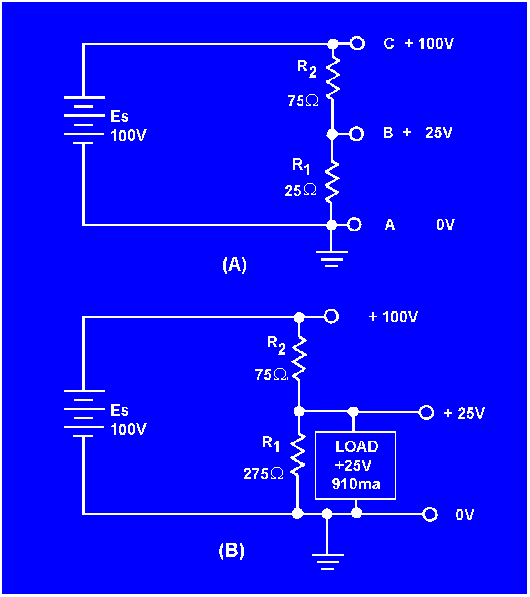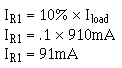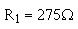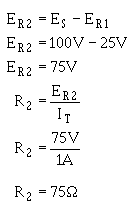power sources. This method is expensive and requires a considerable amount of room. The most common method of supplying these voltages is to use a single voltage source and a VOLTAGE DlVlDER."> Voltage dividersCustom SearchEffects of open and short circuitsMatter, Energy, and ElectricityMultiple-load voltage dividersVOLTAGE DIVIDERS

Most electrical and electronics equipment use voltages of various levels throughout their circuitry.

One circuit may require a 90-volt supply, another a 150-volt supply, and still another a 180-volt supply. These voltage requirements could be supplied by three individual power sources. This method is expensive and requires a considerable amount of room. The most common method of supplying these voltages is to use a single voltage source and a VOLTAGE DlVlDER. Before voltage dividers are explained, a review of what was discussed earlier concerning voltage References may be of help.

As you know, some circuits are designed to supply both positive and negative voltages. Perhaps now you wonder if a negative voltage has any less potential than a positive voltage. The answer is that 100 volts is 100 volts. Whether it is negative or positive does not affect the feeling you get when you are shocked.

Voltage polarities are considered as being positive or negative in respect to a reference point, usually ground. Figure 3-63 will help to illustrate this point.

Figure 3-63. - Voltage polarities.Figure 3-63(A) shows a series circuit with a voltage source of 100 volts and four 50-ohm resistors connected in series. The ground, or reference point, is connected to one end of resistor R1. The current in this circuit determined by Ohm's law is .5 amp. Each resistor develops (drops) 25 volts. The five tap-off points indicated in the schematic are points at which the voltage can be measured. As indicated on the schematic, the voltage measured at each of the points from point A to point E starts at zero volts and becomes more positive in 25 volt steps to a value of positive 100 volts.

In figure 3-63(B), the ground, or reference point has been moved to point B. The current in the circuit is still .5 amp and each resistor still develops 25 volts. The total voltage developed in the circuit remains at 100 volts, but because the reference point has been changed, the voltage at point A is negative 25 volts. Point E, which was at positive 100 volts in figure 3-63(A), now has a voltage of positive 75 volts. As you can see the voltage at any point in the circuit is dependent on three factors; the current through the resistor, the ohmic value of the resistor, and the reference point in the circuit.

A typical voltage divider consists of two or more resistors connected in series across a source voltage (Es). The source voltage must be as high or higher than any voltage developed by the voltage divider. As the source voltage is dropped in successive steps through the series resistors, any desired portion of the source voltage may be "tapped off" to supply individual voltage requirements. The values of the series resistors used in the voltage divider are determined by the voltage and current requirements of the loads.

Figure 3-64 is used to illustrate the development of a simple voltage divider. The requirement for this voltage divider is to provide a voltage of 25 volts and a current of 910 milliamps to the load from a source voltage of 100 volts. Figure 3-64(A) provides a circuit in which 25 volts is available at point B. If the load was connected between point B and ground, you might think that the load would be supplied with 25 volts. This is not true since the load connected between point B and ground forms a parallel network of the load and resistor R1. (Remember that the value of resistance of a parallel network is always less than the value of the smallest resistor in the network.)

Figure 3-64. - Simple voltage divider.Since the resistance of the network would now be less than 25 ohms, the voltage at point B would be less than 25 volts. This would not satisfy the requirement of the load.

To determine the size of resistor used in the voltage divider, a rule-of-thumb is used. The current in the divider resistor should equal approximately 10 percent of the load current. This current, which does not flow through any of the load devices, is called bleeder current.

Given this information, the voltage divider can be designed using the following steps.

Determine the load requirement and the available voltage source.Select bleeder current by applying the 10% rule-of-thumb.Calculate bleeder resistance.The value of R1 may be rounded off to 275 ohms:Calculate the total current (load plus bleeder).Calculate the resistance of the other divider resistor(s).The voltage divider circuit can now be drawn as shown in figure 3-64(B).

What information must be known to determine the component values for a voltage divider?

If a voltage divider is required for a load that will use 450 mA of current, what should be the value of bleeder current?

If the load in question 50 requires a voltage of +90 V, what should be the value of the bleeder resistor?

If the source voltage for the voltage divider in question 50 supplies 150 volts, what is the total current through the voltage divider?Integrated Publishing, Inc. - A (SDVOSB) Service Disabled Veteran Owned Small Business